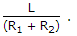# Electronics and Communication Engineering - Networks Analysis and Synthesis

1.

An R-L series circuit has R = 5 Ω and L = .01 H. It is fed by ac 50 Hz supply. The rms current in the circuit is 5 A. The active power supplied by the source is

 A. 25 W B. 50 W C. 125 W D. 250 W

Explanation:

P = I2R = 52(5) = 125 W.

2.

A particular current is made up of two components 10A dc and sinusoidal current of peak value 14.14A. The average of resultant current is

 A. zero B. 24.14A C. 10A D. 14.14A

Explanation:

The average of sinusoid over 1 cycle is zero.

3.

In figure, the switch is closed at t = 0. After some time i = 6A and rate of change of current was 4A/s. The value of inductor is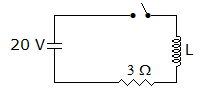A. 1 H B. 1.5 H C. indeterminate D. 0.5 H

Explanation:

Steady state current =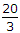A. Therefore, i =(1 - e-3t/L) = 6A and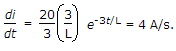From these equations L = 0.5H.

4.

Assertion (A): In series R-L-C circuit, the current may lead or lag the voltage depending on the value of R.

Reason (R): Phase angle of an R-L series circuit is tan-1 (ωL/R).

 A. Both A and R are true and R is correct explanation of A B. Both A and R are true and R is not the correct explanation of A C. A is true but R is false D. A is false but R is true

Explanation:

The current may lead or lag the voltage depending on values of L and C.

5.

In figure the switch has been in position 1 for a long time. At t = 0, the switch is moved to position 2. The equation for transient current is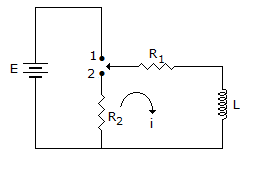A.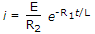B.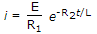C.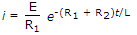D.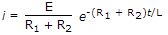When switch is in position 2, time constant is